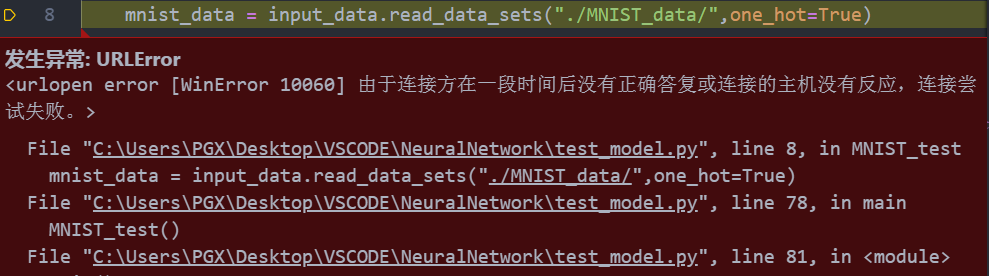# MNIST简单介绍

MNIST是一组经过预处理的手写数字图片数据集，其中每个样本都是一张长28、宽28的灰度图片，其中包含一个0-9的数字。

# 代码块

from tensorflow.examples.tutorials.mnist import input_data
import tensorflow as tf

def MNIST_test():
#加载数据
#获取训练数据
train_data = mnist_data.train
#获取验证数据
validation_data = mnist_data.validation
#获取测试数据
test_data = mnist_data.test
#定义输入变量X
#每个样本的输入是784维向量：一张图片有28*28=784个像素点，每个点用一个浮点数表示其亮度；
X = tf.placeholder(tf.float32,shape=[None,784])
#为它指定一个[None，784]的形状，其中784是单个扁平28乘28像素MNIST图像的维数，
#无表示第一个维度，对应于批量大小，可以任何大小

#定义权重
w = tf.Variable(tf.zeros([784,10],dtype=tf.float32))
#定义偏置
b = tf.Variable(tf.zeros(),dtype=tf.float32)
#计算输出y
#输出是10维向量，十个分量分别表示输入图中数字是0~9的可能性，其中可能性最大的
Y = tf.nn.softmax(tf.matmul(X,w)+b)

#定义预测时的输出
Y_ = tf.placeholder(tf.float32,[None,10])
#定义损失函数
loss_func = tf.reduce_mean(-tf.reduce_sum(Y_ * tf.log(Y)))
#定义训练时候的优化函数

#变量初始化
init = tf.global_variables_initializer()

with tf.Session() as sess:
sess.run(init)

for i in range(10000):
#每次随机选择100个样本进行训练
batch_xs,batch_ys = train_data.next_batch(100)
train_step.run({X:batch_xs,Y_:batch_ys})

#计算准确率
correct_pred = tf.equal(tf.argmax(Y,1),tf.argmax(Y_,1))
accuracy = tf.reduce_mean(tf.cast(correct_pred,tf.float32))
#计算模型在训练集上的准确率
print("train accray:%.4f" % accuracy.eval({X:train_data.images,Y_:train_data.labels}))
#计算模型在测试集上的准确率
print("test accuracy:%.4f" % accuracy.eval({X:test_data.images,Y_:test_data.labels}))

def main():
MNIST_test()

if __name__ == "__main__":
main()


train accray:0.9332
test accuracy:0.9235


# 解决问题：# 代码剖析：

tf.placeholder(tf.float32,shape=[None,784])


loss_func = tf.reduce_mean(-tf.reduce_sum(Y_ * tf.log(Y),axis=1))


tf.reduce_mean 函数用于计算张量tensor沿着指定的数轴（tensor的某一维度）上的的平均值，主要用作降维或者计算tensor（图像）的平均值。
axis=0：列；axis=1：行
reduce_sum() 用于计算张量tensor沿着某一维度的和，可以在求和后降维

correct_pred = tf.equal(tf.argmax(Y,1),tf.argmax(Y_,1))
accuracy = tf.reduce_mean(tf.cast(correct_pred,tf.float32))


tf.argmax 函数能给出某个tensor对象在某一维上的其数据最大值所在的索引值。由于标签向量是由0,1组成，因此最大值1所在的索引位置就是类别标签。用 tf.equal 来检测我们的预测是否真实标签匹配(索引位置一样表示匹配)

tf.cast()函数的作用是执行 tensorflow 中张量数据类型转换
tf.cast()数据类型转换

# 参考学习传送门：

©️2019 CSDN 皮肤主题: 1024 设计师: 上身试试